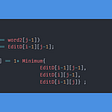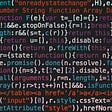# C++ PROGRAM TO REVERSE THE NUMBER!!!

|LOGIC OF REVERSING THE NUMBER|

In this program, we are getting numbers as input from the user and reversing that number.

• Let’s start the program with a header file.

“#include<iostream>” →Input-output continuous bytes of data.

“using namespace std;” →used to store variable name.

“int main()” →returns datatype function.”

“{

int n, reverse=0, rem;

cout<<” Enter a number: “;

cin>>n;”

• Let us declare the variable in integer form (n, reverse=0, rem) because we going to enter numbers; In “cout statement”, we have given enter a number.

cout →character output.

cin →character input.

“while(n>0)

{rem=n%10;

reverse=reverse*10+rem;

n/=10;}”

# Step 1 — Isolate the last digit in number

`lastDigit = number % 10`

The modulo operator (%) returns the remainder of a division. In this case, we divide the number by 10 and return the remainder. Consider the integer 1234. The tens column (30) is divided by 10 without remainder, the hundreds column (200) as well, and also the thousands column (1000). The remainder of the division will be 4 representing the ones column that could not be divided by 10. We now have the means to isolate the last digit of any integer. We save this in the variable the last digit.

# Step 2 — Append the last digit to reverse

`reverse = (reverse * 10) + lastDigit`

We can start building the reversed number now by appending last digit onto the end of a reverse. Remember that both of these variables are integers so we can not simply concatenate. We multiply reverse by 10 so that the ones column becomes the tens column, the tens column becomes the hundreds column, and so on. This also leaves us with a new ones column where we can add our last digit which we determined was 4 in our example.

reverse = (0 * 10) + 4

reverse = 4

We have successfully copied the last digit of the number and appended it to reverse.

# Step 3-Remove last digit from the number

`number = number / 10`

Notice that number is still 1234, even though we have already used that 4 on the end. To remove the last digit from a number we simply divide it by 10. This works because we are performing integer division which rounds results down to the nearest integer (ex. 244 / 10 = 24).

# Step 4-Iterate this process

`while (number > 0)`

Repeat this process until a number is reduced to zero and the reverse is completed.

“cout<<” Reversed Number: “<<reverse<<endl;

return 0;}”

It will reverse a number in output because we have given reverse number in “cout statement”

endl →avoid buffer statement and display in new line.

return 0 →that means the program is executed successfully.reverse number.

# https://www.guvi.in/

--

--

--

## More from Gomathi Keerthana.R.S.

Love podcasts or audiobooks? Learn on the go with our new app.

## Edit Distance## Top 10 tools to work efficiently with remote development team.## The Seven Wonders of MITH Cash V2: Day 2 — Time-Weighted Boardroom Rewards, and Single Asset MIS…## Practical SOLID in Golang: Dependency Inversion Principle## (macOS/OS X) Internet Recovery and how to be NOT scared of it…## How Many Threads is Too Many?## The Cutting Edge## Compilation Process for C Programs, how does it works?!